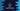# C program to explain how fmod and modf function works### C program to explain how fmod and modf works :

In this C programming tutorial, we will learn how fmod and modf function works, what arguments these functions take and what are the return values. Function fmod is defined as :

``double fmod(double x,double y)``

Similarly, the function modf is defined as :

``double modf(double x,double *y)``

Now, let’s see how fmod works : double fmod(double x,double y) takes two double variables x and y and returns the remainder found by dividing x by y. Similarly, the function double modf(double x,double *y) divides a number into its integer and fraction parts.Let’s take a look at the program to understand both of these functions :

### C program :

``````#include<stdio.h>
#include<math.h>

int main(){
//1
double firstNumber;
double secondNumber;

//2
double number;
double fractionPart;
double intPart;

//3
printf("Enter the first number : ");
scanf("%lf",&firstNumber);

//4
printf("Enter the second number : ");
scanf("%lf",&secondNumber);

//5
printf("fmod(firstNumber,secondNumber) is %lf \n",fmod(firstNumber,secondNumber));

//6
printf("Enter another number : ");
scanf("%lf",&number);

//7
fractionPart = modf(number,&intPart);
printf("Interger component : %lf\n",intPart);
printf("Fraction component : %lf\n",fractionPart);
}``````

### Explanation :

1. Create two double variables to store two numbers.
2. Create three more double variables to store the number, its integer part, and its fraction part.
3. Ask the user to enter the first number. Read it and store it in firstNumber variable.
4. Similarly, ask the user to enter the second number, read and store it in secondNumber variable.
5. Print the fmod value of the firstNumber and secondNumber.
6. Ask the user to enter another number for modf. Read and store it in number variable.
7. Find out the fractionPart by passing the number and address of intPart to the modf function.It will store the integer part in intPart variable.
8. Print both integer and fraction component of the number.

### Sample Output :

``````Enter the first number : 12
Enter the second number : 5
fmod(firstNumber,secondNumber) is 2.000000
Enter another number : 12.334
Interger component : 12.000000
Fraction component : 0.334000``````